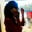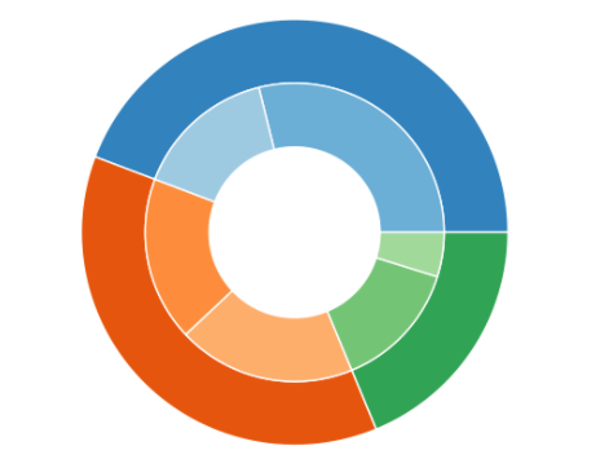Related Tags

python
community creator

# How to make a nested pie chart in PythonSmriti Mallick

A nested pie chart, also known as nested donut chart, displays the given data in multiple levels. Here, we’re going to understand how to implement a nested pie chart using matplotlib.

## What is Matplotlib?

Matplotlib is a plotting library in Python, and its numerical mathematics extension NumPy, that allows users to create static, animated, and interactive visuals.

### Implementing nested pie chart

First, we’re going to import the required libraries using the import function:

import numpy as np
import pandas as pd
import matplotlib.pyplot as plt


Next, we’ll assign values to the wedges of the pie chart. Here, the nested pie takes values in three different categories. We’re going to assign data corresponding to three classes.

In the inner circle, we will represent value as a member of its own group in the form of percentages. In the outer circle, we’ll represent their original categories along with the percentages.

After plotting the Pie, the donut shape should look like:### Code

Here, we take scores of three students and plot a nested pie chart using the percentage of these scores.

To achieve the concentric shape, we set the width of pie with the wedgeprops function.

To use data specific columns from the given data, we use df.iloc[] – this allows us to take certain values from the list.

# Importing required libraries
import numpy as np
import pandas as pd
import matplotlib.pyplot as plt

# Taking raw data of three students
source_data={'students':['Jake','Amy','Boyle'],
'math_score':[68,82,97],
'english_score':[70,93,99],
'physics_score':[73,85,95]}

# Segregating the raw data into usuable form
df=pd.DataFrame(source_data,columns=
['students','math_score','english_score','physics_score'])
df['cumulative_score']=df['math_score']+df['english_score']
+df['physics_score']

# Seperating the sub-parts of the given data
x1= df.iloc[0:3,1]
x2= df.iloc[0:3,2]

# Setting figure colors
cmap = plt.get_cmap("tab20c")
outer_colors = cmap(np.arange(3)*4)
inner_colors = cmap(np.array([1,5,9]))

# Setting the size of the figure
plt.figure(figsize=(8,6))

# Plotting the outer pie
plt.pie(x1, labels = df.iloc[0:3, 0],
startangle=90, pctdistance =0.88 ,colors=outer_colors,
autopct = '%1.1f%%', radius= 1.0, labeldistance=1.05,
textprops ={ 'fontweight': 'bold','fontsize':13},
wedgeprops = {'linewidth' : 3, 'edgecolor' : "w" } )

# PLotting the inner pie
plt.pie(x2,startangle=90, pctdistance =0.85,colors=inner_colors,
textprops ={'fontweight': 'bold' ,'fontsize':13},
wedgeprops = {'linewidth' : 3, 'edgecolor' : "w" } )

# Creating the donut shape for the pie
centre_circle = plt.Circle((0,0), 0.25, fc='white')
fig= plt.gcf()

# Plotting the pie
plt.axis('equal')  # equal aspect ratio
plt.legend( loc=3, fontsize =15)
plt.tight_layout()
plt.show()

The above code plots a nested pie chart, which gives us the percentages or marks scored by different students. You can add more layers to the pie by adding more data columns.

RELATED TAGS

python
community creator

CONTRIBUTORSmriti Mallick
RELATED COURSES

View all Courses

Keep Exploring

Learn in-demand tech skills in half the time